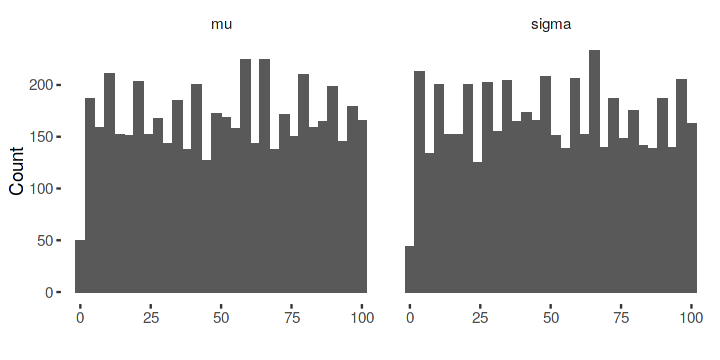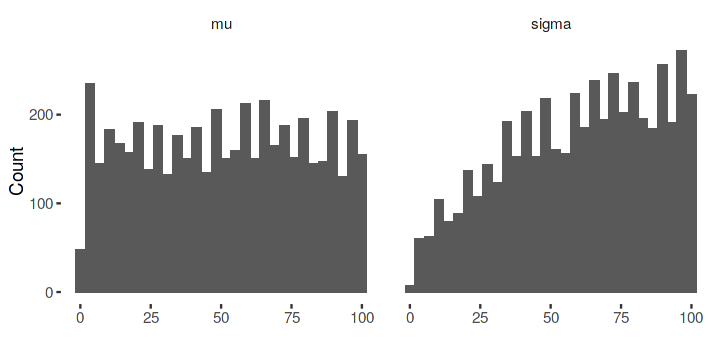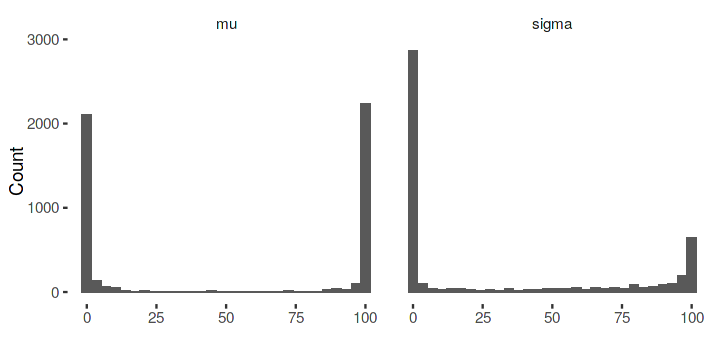# Simulation-based calibration¶

Simulation-based calibration (SBC) is a method for visually validating Bayesian inferences. SBC is useful for detection of either misspecified models, inaccurate computation or bugs in the implementation of a probabilistic program. So SBC is not a validation for the inference itself, but the technical aspect of the program.

Consider a generative model:

\begin{align} \tilde{\theta} & \sim P(\theta) \\ \tilde{y} & \sim P(y \mid \tilde{\theta}) \\ \{ \theta_1, \dots, \theta_L \} & \sim P(\theta \mid \tilde{y}) \end{align}

The rank of a prior sample $\tilde{\theta}$ in comparison to an exact posterior sample $\{ \theta_1, \dots, \theta_L \}$:

\begin{align} r(\{ \theta_1, \dots, \theta_L \}, \tilde{\theta}) = \sum_l^L \mathbb{I}(\theta_l < \tilde{\theta}) + 1 \end{align}

is a discrete-uniform random variable in $[1, L + 1 ]$ (see the original paper for a proof). Thus we can use this as a testing procedure if our inferences work.

We follow Algorithm 2 from the original paper and implement SBC in Stan.

In :
library(rstan)
suppressMessages(library(tidyverse))
options(repr.plot.width = 6, repr.plot.height = 3)

Loading required package: ggplot2
rstan (Version 2.18.2, GitRev: 2e1f913d3ca3)
For execution on a local, multicore CPU with excess RAM we recommend calling
options(mc.cores = parallel::detectCores()).
To avoid recompilation of unchanged Stan programs, we recommend calling
rstan_options(auto_write = TRUE)


SBC is essentially pretty simple. For $N$ iterations we run a while loop to create a posterior sample that has at least a target effective sample size $n_{eff}$ (which is set by us). We do so by resampling and thinning until the posterior sample for every iteration reaches the target $n_{eff}$ (within the while loop). Then we compute the rank for every parameter using the sum as defined above. That is it. If the inference worked, the ranks are uniformly distributed.

In :
sbc <- function(model, data)
{
ranks <- matrix(0, N, 2, dimnames = list(NULL, c("mu", "sigma")))
for (n in seq(N))
{
thin <- init_thin
while (thin < max_thin)
{
fit <- suppressWarnings(
sampling(model, data = data,
chains = 1, iter = 2 * thin * L,
thin = thin, control = list(adapt_delta = 0.99), refresh = 0)
)
n_eff <- summary(fit)$summary["lp__", "n_eff"] if (n_eff >= target_neff) break; thin <- 2 * thin } ranks[n,] <- apply(rstan::extract(fit)$idsim, 2, sum) + 1
}
ranks
}


We start with a simple example where we set two parameters, generate data from them and then compare the posterior to these parameters. The comparison is done in the generated quantities block.

In :
model.file <- "_models/sbc_1.stan"

data {
int<lower=1> n;
vector[n] x;
}

transformed data {
real beta_sim = normal_rng(0, 1);
real<lower=0> sigma_sim = lognormal_rng(0, 1);

vector[n] y_sim;
for (i in 1:n)
y_sim[i] = normal_rng(x[i] * beta_sim, sigma_sim);
}

parameters {
real beta;
real<lower = 0> sigma;
}

model {
beta ~ normal(0, 1);
sigma ~ lognormal(0, 1);
y_sim ~ normal(x * beta, sigma);
}

generated quantities {
int idsim = { beta < beta_sim, sigma < sigma_sim };
}

In :
model <- stan_model(model.file)

In :
?sampling


We run the loop $5000$ times and sample $100$ times. We also set some other parameters that Stan or SBC needs, such as init_thin which specifies the period for saving samples or target_neff which is the effective sample size we want to have.

In :
N <- 5000
L <- 100
init_thin <- 1
max_thin <- 64
target_neff <- .8 * L


We also define a histogram plotting method for the ranks.

In :
plot_fit <- function(fit)
{
as.data.frame(fit) %>%
tidyr::gather("param", "value") %>%
ggplot(aes(value)) +
geom_histogram(bins=30) +
scale_y_continuous("Count") +
scale_x_continuous("") +
facet_grid(. ~ param) +
ggthemes::theme_tufte()
}


Then we run SBC and plot the ranks. We create some artifical data for the model first.

In :
n <- 10
x <- rnorm(n)

fit <- sbc(model, list(x=x, n=n))
plot_fit(fit)Given the low number of trials, the histogram looks fairly uniform (as expected since the model was specified correctly).

Next we test some pathological cases to see if the ranks change with mis-specified models.

In :
model.file <- "_models/sbc_2.stan"

data {
int<lower=1> n;
vector[n] x;
}

transformed data {
real beta_sim = normal_rng(0, 1);
real<lower=0> sigma_sim = lognormal_rng(0, 1);

vector[n] y_sim;
for (i in 1:n)
y_sim[i] = normal_rng(x[i] * beta_sim, sigma_sim);
}

parameters {
real beta;
real<lower = 0> sigma;
}

model {
mu ~ normal(0, 10);
sigma ~ lognormal(0, 5);
y_sim ~ student_t(10, x * beta, sigma);
}

generated quantities {
int idsim = { beta < beta_sim, sigma < sigma_sim };
}

In :
model <- stan_model(model.file)
fit <- sbc(model, list(x=x, n=n))
plot_fit(fit)And another pathological example:

In :
model.file <- "_models/sbc_3.stan"

data {
int<lower=1> n;
vector[n] x;
}

transformed data {
real beta_sim = normal_rng(0, 11);
real<lower=0> sigma_sim = lognormal_rng(0, 10);

vector[n] y_sim;
for (i in 1:n)
y_sim[i] = student_t_rng(10, beta_sim, sigma_sim);
}

parameters {
real beta;
real<lower = 0> sigma;
}

model {
beta ~ normal(0, 1);
sigma ~ lognormal(0, 1);
y_sim ~ normal(x * beta, sigma);
}

generated quantities {
int idsim = { beta < beta_sim, sigma < sigma_sim };
}

In :
model <- stan_model(model.file)
fit <- sbc(model, list(x=x, n=n))
plot_fit(fit)